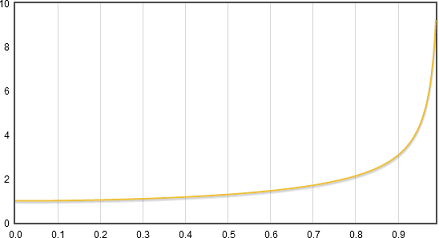# E=mc2

### Basic Concepts and principles

It is a fact the conservation of momentum, for example when we play billiards and our ball strikes others, the sum value of momentums must be conserved, ie if we call respectively $\vec{p}, ;\ \vec{p'}$ to linear momentums before and after collision of the ball you have to

$\sum_{i}\vec{p} = \sum_{i}\vec{p}' \; \Leftrightarrow \; \sum_{i}m_i\vec{v_i} = \sum_{i}m_i\vec{v_i}'$

We'll see how, starting from these concepts (slightly redefined in special relativity) and with math level high school student it is possible to obtain the most famous formula of science

### Extended Theory

In the theory of special relativity is replaced the velocity by four-velocity and the linear momentum by por el four-momentum .

Given a material particle moving with constant velocity v = (v1, v2, v3), then it is holds (x, y z) = vt and its universe line must be

$\alpha(\tau)=(t(\tau), \vec{x}(\tau))= (t(\tau), \vec{v}t(\tau))$

To satisfy that α' be future and norm be 1 it is necessary

$\displaystyle {\frac{dt}{d\tau}=\frac{1}{\sqrt{1-v^2}}=\gamma}$.

So

$\vec{V}=\gamma(1,v_1,v_2,v_3)$

$\vec{P} = m \vec{V}= m\gamma(1,v_1,v_2,v_3)$

as stated $\displaystyle {\gamma =\frac{1}{\sqrt{1-v^2}}}$

Note that four-momentum and four-velocity almost coincide in their last 3 coordinates with their respective analogues of classical mechanics, velocity and momentum respectively, but with a small modification factor (multiplication by γ) wich appears at Lorentz Transformation section.

In fact the last 3 coordinates of $\vec{P}$ are called 3-momentum and we denote them by p.

The first coordinate of $\vec{P}$ is called Energy and we denote it by E, therefore.

$\vec{P} = (E, \vec{p}$, con $E=\gamma m$ y $\vec{p}=\gamma \vec{v}$

From here it follows that

$E= (m^2+||\vec{p}||^2)^{\frac{1}{2}}$

In non-relativistic units it is

$E= c(m^2 c^2 + ||\vec{p}||^2)^{\frac{1}{2}}$

Making $\vec{v} = 0$ it is follows

$E= m c^2$Enegy growing with speed increases in relativistic units. Note as Energy makes itself infinite when velocity tends to finite value of speed of ligth

Note that γ is same γ of Lorentz Transformation (now expressed in relativistic units) and the value of E increasing as velocity increasing is

E → ∞ when||v|| → 1+

From here, it follows that when a material particle accelerates and increases its velocity to speed of light, its mass (and therefore its energy) tends to become infinite, hence it is not possible to accelerate a particle at the speed of light, because for this we need infinite energy.

With a considerable expenditure of energy we could accelerate a spaceship to 90% of speed of light, would be a good number, but we could never get to fully cover the 10% missing.

# Was useful? want add anything?

Post here

### Post from other users

Post here